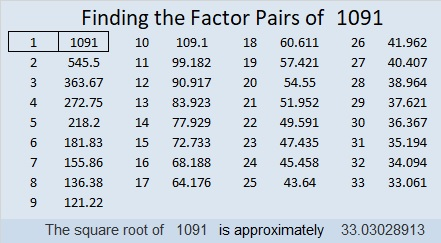# 1091 and Level 5

Can you figure out where to put all the numbers from 1 to 10 in both the first column and the top row so that those factors and the clues can become a multiplication table? Some of the clues might be a little tricky, but I’m sure you can figure them all out.Print the puzzles or type the solution in this excel file: 10-factors-1087-1094

Here are a few facts about the number 1091:

• 1091 is a prime number.
• Prime factorization: 1091 is prime.
• The exponent of prime number 1091 is 1. Adding 1 to that exponent we get (1 + 1) = 2. Therefore 1091 has exactly 2 factors.
• Factors of 1091: 1, 1091
• Factor pairs: 1091 = 1 × 1091
• 1091 has no square factors that allow its square root to be simplified. √1091 ≈ 33.03029

How do we know that 1091 is a prime number? If 1091 were not a prime number, then it would be divisible by at least one prime number less than or equal to √1091 ≈ 33. Since 1091 cannot be divided evenly by 2, 3, 5, 7, 11, 13, 17, 19, 23, 29 or 31, we know that 1091 is a prime number.1091 is the first prime number in the prime triplet (1091, 1093, 1097). It is also the middle number in the prime triplet (1087, 1091, 1093).

1091 looks interesting when it is written in some other bases:
It’s 13331 in BASE 5 because 1(5⁴) + 3(5³) + 3(5²) + 3(5) + 1(1) = 1091,
3D3 in BASE 17 (D is 13 base 10) because 3(17²) + 13(17) + 3(1) = 1091,
and it’s 123 in BASE 32 because 1(32²) + 2(32) + 3(1) = 1091